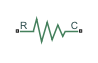# Nonlinear Translational Spring

Translational spring based on polynomial or table lookup parameterizations

•Libraries:
Simscape / Driveline / Couplings & Drives / Springs & Dampers

## Description

The Nonlinear Translational Spring block represents a translational spring with a nonlinear force-displacement curve. The spring force magnitude is a general function of displacement and does not necessarily need to satisfy Hooke’s law. You can parameterize the force-displacement relationship using a polynomial or a lookup table. The spring force can be symmetric or asymmetric with respect to zero deformation.

The symmetric polynomial parameterization defines spring force according to the expression

`$F={k}_{1}x+sign\left(x\right)\cdot {k}_{2}{x}^{2}+{k}_{3}{x}^{3}+sign\left(x\right)\cdot {k}_{4}{x}^{4}+{k}_{5}{x}^{5},$`

where:

• F is the spring force.

• k1, k2, ..., k5 are the spring coefficients.

• x is the relative displacement between ports R and C.

• xinit is the initial relative displacement.

At simulation start x is equal to xinit.

To avoid zero-crossings that slow simulation, eliminate the sign function from the polynomial expression by specifying an odd polynomial (b2,b4 = 0).

The asymmetric polynomial parameterization defines spring force according to the expression:

`$F=\left\{\begin{array}{cc}{k}_{1t}x+{k}_{2t}{x}^{2}+{k}_{3t}{x}^{3}+{k}_{4t}{x}^{4}+{k}_{5t}{x}^{5},& x\ge 0\\ {k}_{1c}x+{k}_{2c}{x}^{2}+{k}_{3c}{x}^{3}+{k}_{4c}{x}^{4}+{k}_{5c}{x}^{5},& x<0\end{array},$`

where:

• k1, k2, ..., k5 are the spring tension coefficients.

• k1, k2, ..., k5 are the spring compression coefficients.

Both polynomial parameterizations use a fifth-order polynomial expression. To use a lower-order polynomial, set the unneeded higher-order coefficients to zero. To use a higher-order polynomial, fit to a lower-order polynomial or use the table lookup parameterization.

Setting Parameterization to `By table lookup` defines the damping torque based on a set of torque and angular velocity vectors. If you do not enter a datapoint for the origin, the block automatically adds one (zero angular velocity and zero torque).

### Variables

To set the priority and initial target values for the block variables prior to simulation, use the Initial Targets section in the block dialog box or Property Inspector. For more information, see Set Priority and Initial Target for Block Variables.

Nominal values provide a way to specify the expected magnitude of a variable in a model. Using system scaling based on nominal values increases the simulation robustness. Nominal values can come from different sources, one of which is the Nominal Values section in the block dialog box or Property Inspector. For more information, see Modify Nominal Values for a Block Variable.

## Ports

### Conserving

expand all

Mechanical rotational conserving port associated with the follower end.

Mechanical rotational conserving port associated with the base end.

## Parameters

expand all

Spring behavior selection. You can choose to parameterize by polynomial or lookup table.

Spring behavior symmetry about the point of zero deformation. When you select `Symmetric`, the block applies one polynomial symmetrically about the point of zero deformation. When you select `Two-sided`, the block applies unique polynomials for tension and compression.

#### Dependencies

To enable this parameter, set Parameterization to `By polynomial`.

Polynomial spring rate coefficients. The highest non zero-order element must be positive. Physical units represent the first coefficient, and the block selects the appropriate higher-order units for you. This vector must have a length of 5.

#### Dependencies

To enable this parameter, set Parameterization to `By polynomial` and Symmetry to `Symmetric`.

Polynomial coefficients for the spring tension. Physical units represent the first coefficient, and the block selects the appropriate higher-order units for you. This vector must have a length of 5.

#### Dependencies

To enable this parameter, set Parameterization to `By polynomial` and Symmetry to `Two-sided`.

Polynomial coefficients for the spring compression. Physical units represent the first coefficient, and the block selects the appropriate higher-order units for you. This vector must have a length of 5.

Relative displacement values with respect to the zero-deformation position for the corresponding elements in the Force vector parameter. This parameter must be the same length as the Force vector parameter. The minimum vector length depends on the interpolation method: two for `Linear` and three for `Smooth`. If not included in the vector, the block automatically adds a point at the origin deformation–force coordinate.

Force values for the corresponding element in the Deformation vector parameter. This parameter must be the same length as the Deformation vector parameter. The minimum vector length is: two for `Linear` and three for `Smooth`. The block automatically adds a point at the origin deformation-force coordinate if you do not specify one.

Lookup table breakpoint interpolation. The block uses the `tablelookup` function to model nonlinearity by using vector data to map input values to output values:

• `Linear` — Select this option for the lowest computational cost.

• `Smooth` — Select this option to produce a continuous curve with continuous first-order derivatives.

For more information, see `tablelookup`.

Lookup table breakpoint extrapolation. This method determines the output value when the input value is outside the range specified in the argument list. The block uses the `tablelookup` function to model nonlinearity by using vector data to map input values to output values:

• `Linear` — Select this option to produce a curve with continuous first-order derivatives in the extrapolation region and at the boundary with the interpolation region.

• `Nearest` — Select this option to produce an extrapolation that does not go above the highest point in the data or below the lowest point in the data.

• `Error` — Select this option to avoid extrapolating when you want your data to be within the table range. If the input signal is outside the range of the table, the simulation stops and generates an error.

For more information, see `tablelookup`.

## Version History

Introduced in R2013a